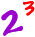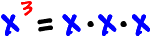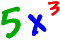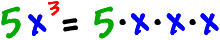Remember from your arithmetic days what this means?That's three 2's all multiplied together:As you know by now, in Pre-Algebra, we work with that unknown guy... Mr. X!

The thing to remember is that x is a number -- we just don't know which one.

So, all the stuff you learned in arithmetic works here too!What if we stick a 5 in front of this thing?The 5 is called a coefficient (because it's a number in front of an x guy) and it's just multiplied in with the x's.)Remember that x is some mystery number...
What would we get if we let x = 3?